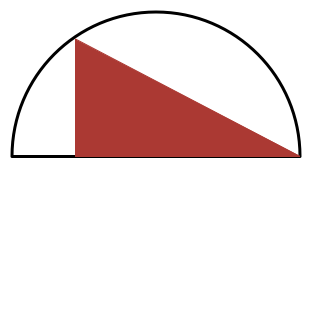Calculus Level 2Let $S$ be the maximum possible area of a right triangle that can be drawn in a semi-circle of radius $1$, where one of the legs (and not the hypotenuse) of the triangle must lie on the diameter of the semicircle.

If $S = \dfrac{a\sqrt{b}}{c},$ where $a,c$ are positive coprime integers and $b$ is a positive square-free integer, find $a + b + c.$

×